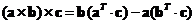## Vector Triple Dot Product

Vector triple dot product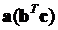is a multiplication of three vectors that produce another vector. First vector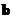and vector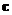are multiplied using inner product , and then the scalar result of the inner product is used as a scalar multiple to the vector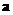. Geometrically triple dot product is the same as vector scalar multiple that stretch or shrink the vectorbased on the angle between vectorand vector. When the vectorand vectorare collinear the angle is zero and the triple dot product exactly the same as vector. As the angle between vectorand vectoris larger than zero, the length of vectorreduces up to zero-vector when the angle between vectorand vectoris perpendicular.

Example
Suppose we have three vectors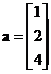,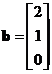and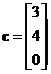.

First we compute dot product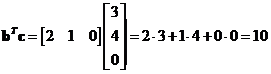Then, the triple dot product is just scalar multiple of the first vectorThe interactive program below helps you to compute vector triple dot algebraically. You input 3 vectors of the same dimension. The program output is the vector triple dot product. Random example button will generate random vectors at the right format.

a ( b . c )

## Properties

Some important properties of related to triple dot product is

• Vector triple dot product is related to triple cross product:
•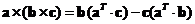•